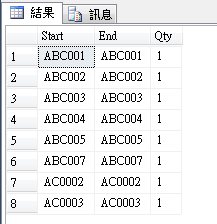#0

## SQL TABLE展開• Start與End欄位資料由前置碼+流水碼組成
• 前置碼僅存在英文與符號
• 前置碼沒有固定長度
• 流水碼僅存在數字
• 流水碼沒有固定長度
• 流水碼不一定從1開始
• 數量反應此行可拆幾組資料

``````DECLARE @TABLE_A AS TABLE
(
[Start] varchar(20),
[End] varchar(20),
[Qty] numeric(10)
)

DECLARE @TABLE_B AS TABLE
(
[Start] varchar(20),
[End] varchar(20),
[Qty] numeric(10)
)

INSERT INTO @TABLE_A VALUES('ABC001','ABC005',5)
INSERT INTO @TABLE_A VALUES('ABC007','ABC007',1)
INSERT INTO @TABLE_A VALUES('AC0002','AC0003',2)
``````

1.避免start和end不一致
2.寫法可以更好寫 不用太多function

### 3 個回答

1

``````DECLARE @TABLE_A AS TABLE
(
[Start] varchar(20),
[End] varchar(20),
[Qty] numeric(10)
)

DECLARE @TABLE_B AS TABLE
(
[Start] varchar(20),
[End] varchar(20),
[Qty] numeric(10)
)

INSERT INTO @TABLE_A VALUES('ABC001','ABC005',5)
INSERT INTO @TABLE_A VALUES('ABC007','ABC007',1)
INSERT INTO @TABLE_A VALUES('AC0002','AC0003',2)

;WITH CTE  AS (
SELECT SUBSTRING([Start],0,PATINDEX('%[0-9]%', [Start])) as prefix,
CAST(SUBSTRING([Start],PATINDEX('%[0-9]%', [Start]),LEN([Start]) - PATINDEX('%[0-9]%', [Start]) + 1) AS INT) fromNum,
CAST(SUBSTRING([End],PATINDEX('%[0-9]%', [End]),LEN([End]) - PATINDEX('%[0-9]%', [End]) + 1) AS INT) toNum,
PATINDEX('%[1-9]%', [Start]) - PATINDEX('%[0-9]%', [Start])  paddingOfZero
FROM @TABLE_A
UNION ALL
SELECT  prefix,
fromNum +1,
toNum,
FROM CTE
WHERE fromNum +1<=toNum
)
INSERT INTO @TABLE_B ([Start],[End],Qty)
1
from cte

SELECT *
FROM @TABLE_B
``````

`Qty`那邊你沒特別說邏輯 我就針對你提問的流水號寫語法,

dbfiddle

https://isdaniel.github.io/CTE-RECURSIVE/

CTE 的寫法好酷，我要花點時間看

``````INSERT INTO @TABLE_B ([Start],[End],Qty)
from CTE
``````

index scan失效似乎會是未來效能上的隱憂...如果考慮到此問題或許還是需要轉後端處理index scan失效似乎會是未來效能上的隱憂...如果考慮到此問題或許還是需要轉後端處理

1

``````DECLARE @TABLE_A AS TABLE
(
[Start] varchar(20),
[End] varchar(20),
[Qty] numeric(10)
)

INSERT INTO @TABLE_A VALUES('ABC001','ABC005',5)
INSERT INTO @TABLE_A VALUES('ABC007','ABC007',1)
INSERT INTO @TABLE_A VALUES('AC0002','AC0003',2)

select left([Start],3) + right('00'+ Convert(varchar,Convert(int,Right([Start],3)) + n-1),3) [Start]
,left([Start],3) + right('00'+ Convert(varchar,Convert(int,Right([Start],3)) + n-1),3) [End]
,1 [Qty]
from @TABLE_A a
,(
select Row_Number()Over(order by number) n
from master..spt_values
) k
where n <= [Qty]
``````0

wwww

x721221 iT邦新手 5 級 ‧ 2021-05-07 13:17:05 檢舉

x721221 iT邦新手 5 級 ‧ 2021-05-07 14:04:46 檢舉

x721221 iT邦新手 5 級 ‧ 2021-05-07 14:06:24 檢舉

x721221 iT邦新手 5 級 ‧ 2021-05-07 14:08:37 檢舉